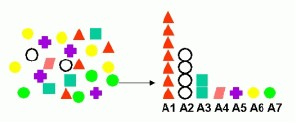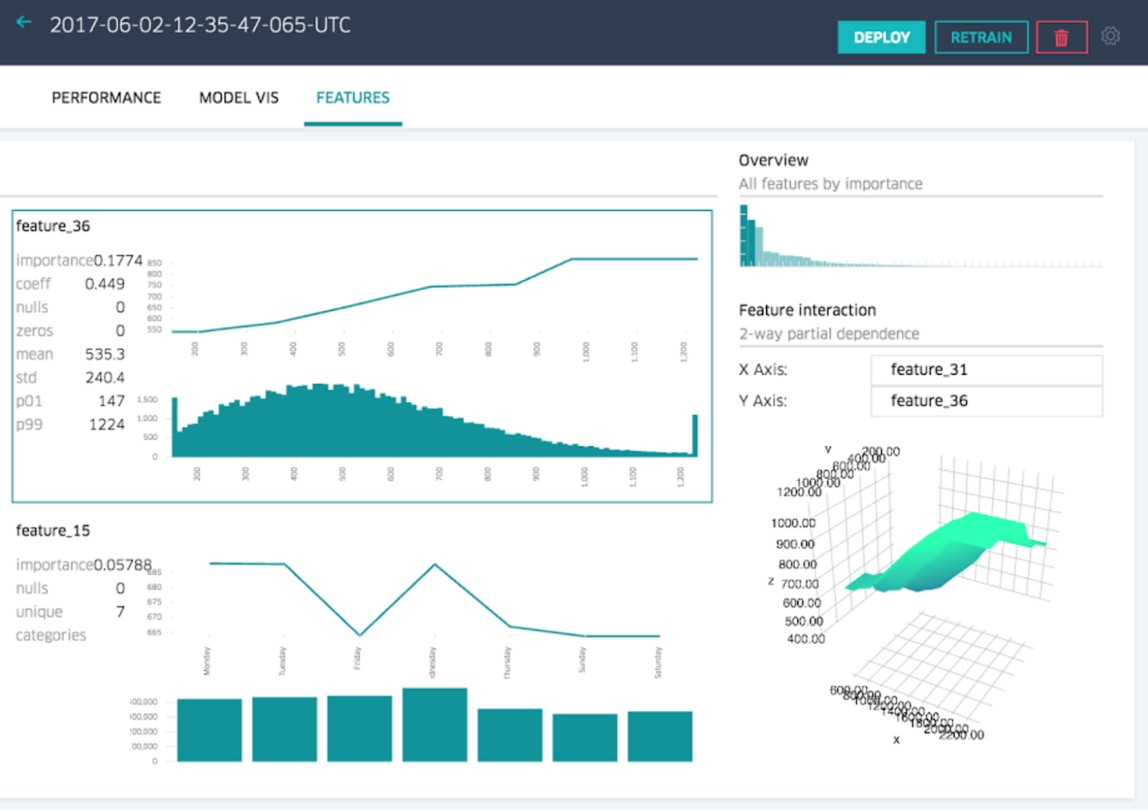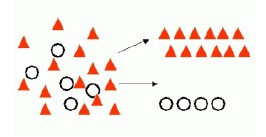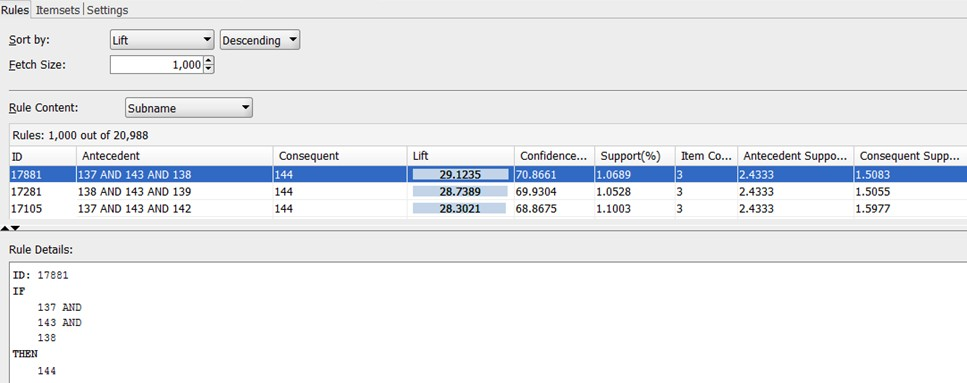# Statistics - Sample (Variable | Attribute | Feature)

A (statistician|data miner) studying a population would be interested in collecting information about different characteristics of the subject (like their length, or weight, or age) in a sample. Those characteristics are called variables.

In a database, they are just columns.

They can take on multiple values. In contrast, a constant has only one value

A variable have:

In mathematics, variables are listed among the arguments that the function takes.

## Data Type

Variables (of an instance) are of two types:

• discrete (called nominal, categorical or qualitative)
• or continuous (called numeric, numerical or quantitative).

and have 4 levels

### Categorical

When a characteristic can be neatly placed into well-defined groups, or categories that do not depend on order, it is called a categorical variable (some statisticians use the word qualitative).

### Numerical

When we are interested in the total number of each species of tortoise, or how many individuals there are per square kilometre. This type of variable is called numerical (or quantitative).

## Usage

Type of attribute Type of model Description
independent variable (predictors|feature) supervised Predictors that affect a given outcome
dependent variable (outcome,…) supervised outcome that are affected by predictors
descriptors (unsupervised|descriptive) Items of information being analysed for natural groupings or associations.

## Variable Name Glossary

Dependent variable Independent variable
Dependent Independent
Outcome Predictor

## Others

### Case id

A Case Id identifies uniquely each record in order to help with model repeatability.

Recommended Pages(Machine|Statistical) Learning - (Predictor|Feature|Regressor|Characteristic) - (Independent|Explanatory) Variable (X)

A Independent variable is a variable used in supervised analysis in order to predict an outcome variable. It's also known as: Predictor Input variable, Regressors, Explanatory variable, CovariateCovariates...(Machine|Statistical) Learning - (Target|Learned|Outcome|Dependent|Response) (Attribute|Variable) (Y|DV)

An (outcome|dependent) variable is ameasure that we want to predict. : the original score collected : the predicted score (or estimator) from the equation. The hat means “estimated” from the...(Mathematics|Statistics) - Statistical Parameter

population parameter A parameter is a numerical characteristic, feature, or measurable factor that help in defining a particular model. Unlike variables, parameters are not listed among the arguments...(Statistics|Machine Learning|Data Mining) - (Unit|Individual|Case|Subject|Observation|Instance|Input)

in Statistics. Each member of a sample is also known as: a unit an individual a case a subject an instance an observation input data Data contains values grouped into variables and observations....(Statistics|Probability|Machine Learning|Data Mining|Data and Knowledge Discovery|Pattern Recognition|Data Science|Data Analysis)

The terms pattern recognition, machine learning, data mining and knowledge discovery in databases (KDD) are hard to separate, as they largely overlap in their scope.Machine Learninsupervised learning methodKDD...Data Mining - Attribute (Importance|Selection) - Affinity Analysis

Attribute importance is a supervised function that identifies and ranks the attributes that are most important in predicting a target attribute. Oracle Data Mining does not support the scoring operation...Data Mining - (Attribute|Feature) (Selection|Importance)

Feature selection is the second class of dimension reduction methods. They are used to reduce the number of predictors used by a model by selecting the best d predictors among the original p predictors....Data Mining - (Classifier|Classification Function)

A classifier is a Supervised function (machine learning tool) where the learned (target) attribute is categorical (“nominal”) in order to classify. It is used after the learning process to classify...Data Mining - (Decision) Rule

Some forms of predictive data mining generate rules that are conditions that imply a given outcome. Rules are if-then-else expressions; they explain the decisions that lead to the prediction. They...Data Mining - (Dimension|Feature) (Reduction)

In machine learning and statistics, dimensionality reduction is the process of reducing the number of random variables (features) under consideration and can be divided into: feature selection (returns...